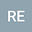Dynamical BLUP modeling with Robertson-Price updating of mean reaction norm parameter values
•## Abstract

A general reaction norm model of any order can be formulated as a linear mixed model. From this follows that estimates of mean phenotypic traits in a population (fixed effects), and predictions of individual additive genetic deviations from mean reaction norm parameter values (random effects), can be found from the best linear unbiased predictions (BLUP) equation in matrix form. The resulting BLUP model is dynamical in the sense that the incidence matrix varies with time. This leads to a straightforward and multivariate alternative to infinite-dimensional and random regression modeling of reaction norms. Based on such a BLUP model, the within-generation changes in predicted mean reaction norm parameter values can be found by use of the Robertson-Price identity, applied on the predicted random effects. From this follows that the between-generation change in the mean values are found from Robertson’s secondary theorem of natural selection, applied on the predicted random effects. This explains why and to which extent the variances of BLUP random effects are underestimated, which is a well-known observation. The dynamical BLUP model will thus produce the mean reaction norms over time, which makes it possible to disentangle the microevolutionary and plasticity components in for example climate change responses. The BLUP responses will depend on the additive genetic relationship matrix A_t. When A_t is an identity matrix, the results will be identical to the results from a variant of the multivariate breeder’s equation, based on the selection gradient with respect to the individual phenotypic trait values. Parameters are assumed to be known and constant, but it is discussed how they can be estimated by means of a prediction error method. Generations are assumed to be non-overlapping, but adjustments for overlapping generations can easily be done.
12 Apr 2023Submitted to Ecology and Evolution
10 May 2023Submission Checks Completed
10 May 2023Assigned to Editor
11 May 2023Review(s) Completed, Editorial Evaluation Pending
14 May 2023Editorial Decision: Revise Minor